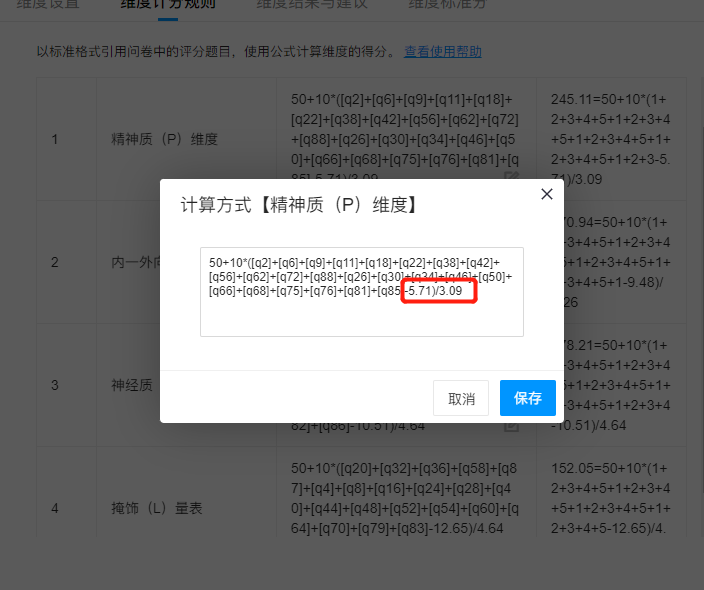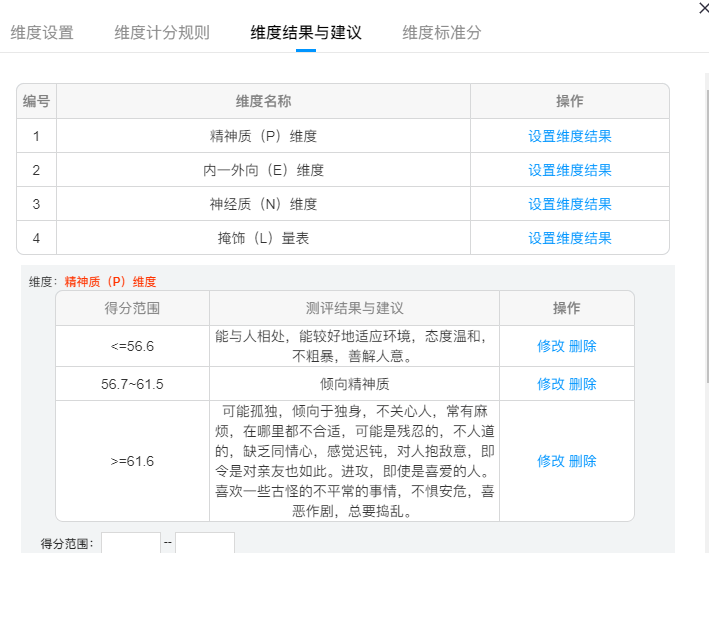# 艾森克人格测试（EPQ）

## 如何配置艾森克人格测评

### 第三步：依据题目调整公式

50+10*([q1]+[q5]+[q10]+[q13]+[q14]+[q17]+[q25]+[q33]+[q37]+[q41]+[q49]+[q53]+[q55]+[q61]+[q65]+[q71]+[q80]+[q84]+[q21]+[q29]+[q45]-9.48)/4.26

50+10*([q3]+[q7]+[q12]+[q15]+[q19]+[q23]+[q27]+[q31]+[q35]+[q39]+[q43]+[q47]+[q51]+[q57]+[q59]+[q63]+[q67]+[q69]+[q73]+[q74]+[q77]+[q78]+[q82]+[q86]-10.51)/4.64

50+10*([q2]+[q6]+[q9]+[q11]+[q18]+[q22]+[q38]+[q42]+[q56]+[q62]+[q72]+[q88]+[q26]+[q30]+[q34]+[q46]+[q50]+[q66]+[q68]+[q75]+[q76]+[q81]+[q85]-5.71)/3.09

50+10*([q20]+[q32]+[q36]+[q58]+[q87]+[q4]+[q8]+[q16]+[q24]+[q28]+[q40]+[q44]+[q48]+[q52]+[q54]+[q60]+[q64]+[q70]+[q79]+[q83]-12.65)/4.64

### 第四步：配置人群样本均数(M)及标准差(SD)### 第五步：维度结果配置